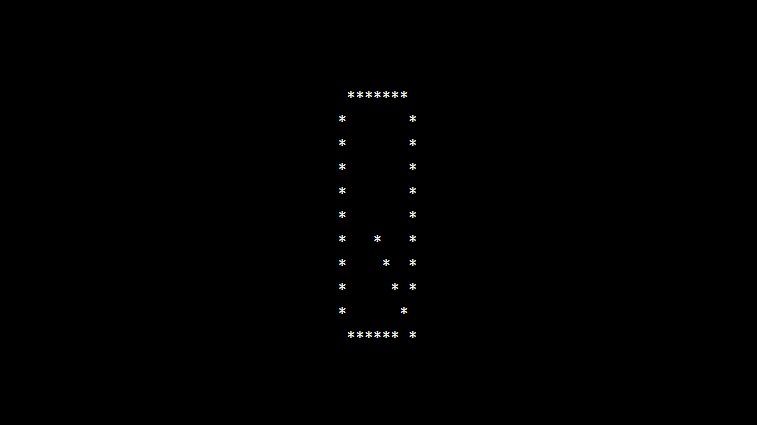# PHP program to print alphabet pattern Q

In this article write a PHP program to print alphabet pattern Q. This Program first takes the numbers of rows and then prints pattern using nested for loops.

Read Also : PHP Program To Print Alphabet Pattern P

## PHP program to print alphabet pattern Q

```<?php
echo "<pre>";
for (\$row = 0; \$row < 11; \$row++) {
for (\$col = 0; \$col <= 11; \$col++) {
if ((\$col == 1 AND \$row != 0 AND \$row != 10) OR (\$row == 0 AND \$col > 1 AND \$col < 9) OR (\$col == 9 AND \$row != 0 AND \$row != 9) OR (\$row == 10 AND \$col > 1 AND \$col < 8) OR (\$col == \$row - 1 AND \$row > 5)) {
echo "*";
} else {
echo "&nbsp;";
}
}
echo "<br>";
}
echo "</pre>";
?>
```

### Output:1 Comment
1. nisha says

alphabet pattern A using star if condition not clear plz explain your code